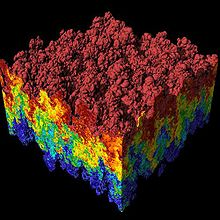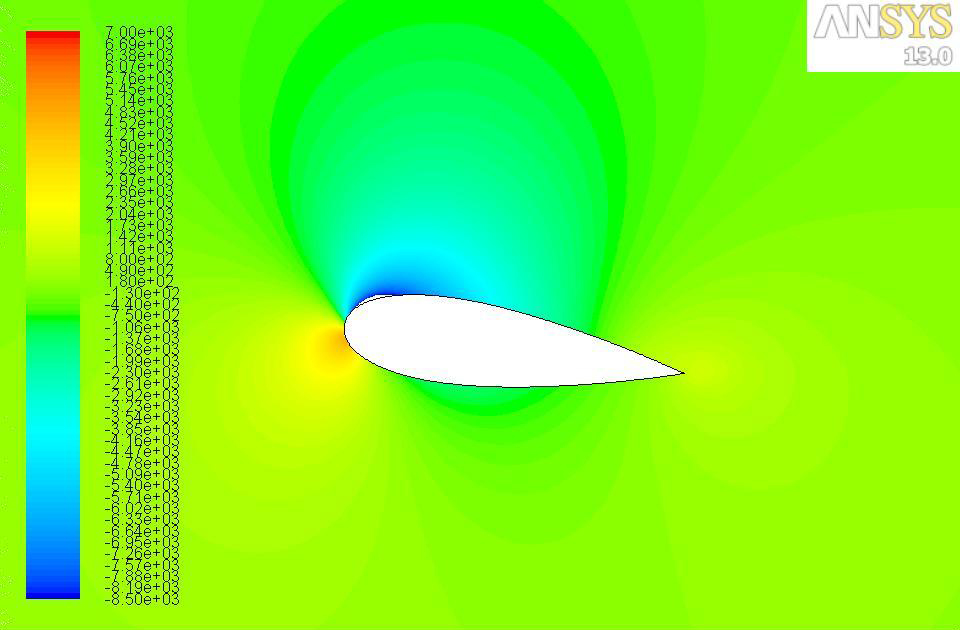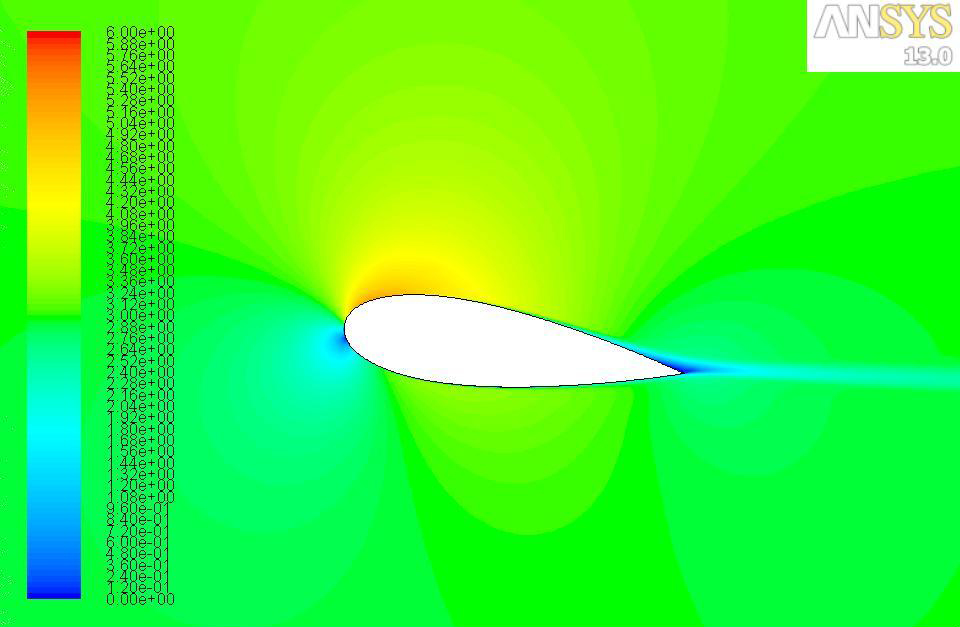HYDROFOIL

## CFD### Computational Fluid Dynamics

Computational Fluid Dynamics (CFD) is a discipline in fluid mechanics that is used to analyse fluid flows with the help of mathematical algorithms and numerical methods. It is based on the famous Navier-Stokes Equations. Complexity of these equations usually demand computers to do the calculations required to simulate the interactions between the fluid layers. As technology advances, better supercomputers are created and more accurate results are achieved. Research continues for transonic, turbulent and even high-hypersonic flows to be modelled for spacecraft and aerospace industry.

### Computational Fluid Dynamics in our Project

Computational Fluid Dynamics (CFD) of the hydrofoil was carried out by our team collaborator Thomas Nevalainen. The main objective was to find dimensions and the angle of attack of the hydrofoil. From here, we could model it and see if we can achieve the required lift force. For this, a generalized lift and drag coefficient were used.Pressure Profile around hydrofoil at 8 degree angle of attackVelocity Profile around hydrofoil at 8 degree angle of attack

### Data used and Results of CFD

• Dimensions
-Chord length= 7 m
-Width= 17 m
• Angle of attack (optimum)= 8 degrees
• Lift force= 385 kN
• Drag force= 10 kN
• Buoyancy= 100 kN
• Coefficient of lift= 0.5
• Coefficient of drag= 0.025
• A pressure profile was also calculated, which was used as part of the structural loading input file for the Finite Element Analysis.